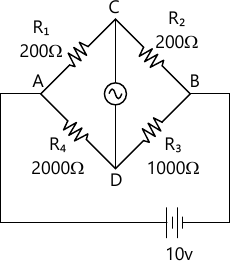MORE IN Electronic Instrumentation
VTU Electronics and Communication Engineering (Semester 3)
Electronic Instrumentation
December 2013
Total marks: --
Total time: --
INSTRUCTIONS
(1) Assume appropriate data and state your reasons
(2) Marks are given to the right of every question
(3) Draw neat diagrams wherever necessary

1 (a) Define the following terms as applied to an instrument:
i) Accuracy and Precision
ii) Random error
iii) Absolute and relative error
iv) Gross error
v) Systematic error.
6 M
1 (b) Determine the value of the multiplier resistance on the 50V range of a DC voltmeter that uses a 250μA meter movement with an internal resistance of 100Ω.
6 M
1 (c) Explain in detail the working of true RMS volt meter and give and give the difference between peak responding and average responding voltmeters.
8 M

2 (a) Explain in detail the working of successive approximation type digital voltmeter and give the O/P for 4 bit control word.
8 M
2 (b) A 41/2 digit DUM is used for voltage measurements, i) Find its resolution ii) How would 67.50V be displayed on a 5V range iii) How would 0.716V be displayed in 1V and 10V ranges.
6 M
2 (c) Explain in detail the working of digital multimeter.
6 M

3 (a) Explain in detail the generation of time based signal for the horizontal deflecting plates.
5 M
3 (b) What is an electronic switch and explain in detail its various modes of operation?
5 M
3 (c) List the various control knobs on the front panel of CRO.
4 M
3 (d) An electrically deflected CRT has a final anode voltage of 2000V and parallel deflecting plates 1.5 cm apart. If the screen is 50 cm from the centre of the deflecting plates, find i) Beam speed ii) Deflection sensitivity of the tube iii) Deflection factor.
6 M

4 (a) Explain in detail the working of sampling oscilloscope with necessary waveforms.
10 M
4 (b) Explain in detail the working of digital storage oscilloscope and list the advantages of DSO.
10 M

5 (a) Explain in detail the working of function generator.
8 M
5 (b) Explain in detail the working of square and pulse generator.
8 M
5 (c) Explain in detail the working of sine and square wave generator.
4 M

6 (a) Explain in detail the working of Wien bridge oscillator and find the parallel 'R' and 'C' that causes a Wien bridge to null with the following components values. R1=2.7 kΩ. R2=22KΩ. C1=5μF, Ra=1000 kΩ and the operating frequency is 2.2 kHz.
12 M
6 (b) For the wheat stone bridge shown in fig Q6(b), the galvanometer has a current sensitivity of 12mm/μA. The internal resistance of galvanometer is 200Ω. Calculate the deflection of the galvanometer caused due to 5Ω unbalance in the arm AD.8 M

7 (a) Explain in detail the working of resistive position transducer and also calculate the output voltage when wiper is 10cm from extreme end for the applied voltage of 5V and if the resistive position transducer uses a shaft with a stroke of 50cm. The total resistance of the potentiometer is 5kΩ.
10 M
7 (b) Explain the construction, principle and operation of LVDT.
10 M

8 (a) Write a short note on signal conditioning system.
6 M
8 (b) Explain the working of piezo electric transducer with circuit diagram.
10 M
8 (c) Compare LED displays and LCD displays.
4 M

More question papers from Electronic Instrumentation